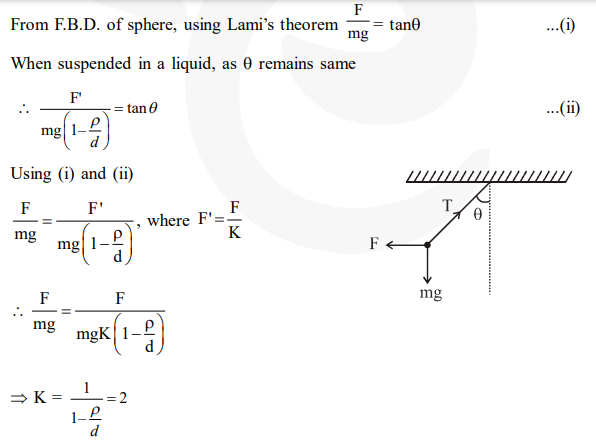# Two identical charged spheres are suspended by strings of equal lengths.

Question:

Two identical charged spheres are suspended by strings of equal lengths. The strings make an angle of $30^{\circ}$ with each other. When suspended in a liquid of density $0.8 \mathrm{~g} \mathrm{~cm}^{-3}$, the angle remains the same. If density of the material of the sphere is $1.6 \mathrm{~g} \mathrm{~cm}^{-3}$, the dielectric constant of the liquid is :

1. 1

2. 4

3. 3

4. 2

Correct Option: , 4

Solution: